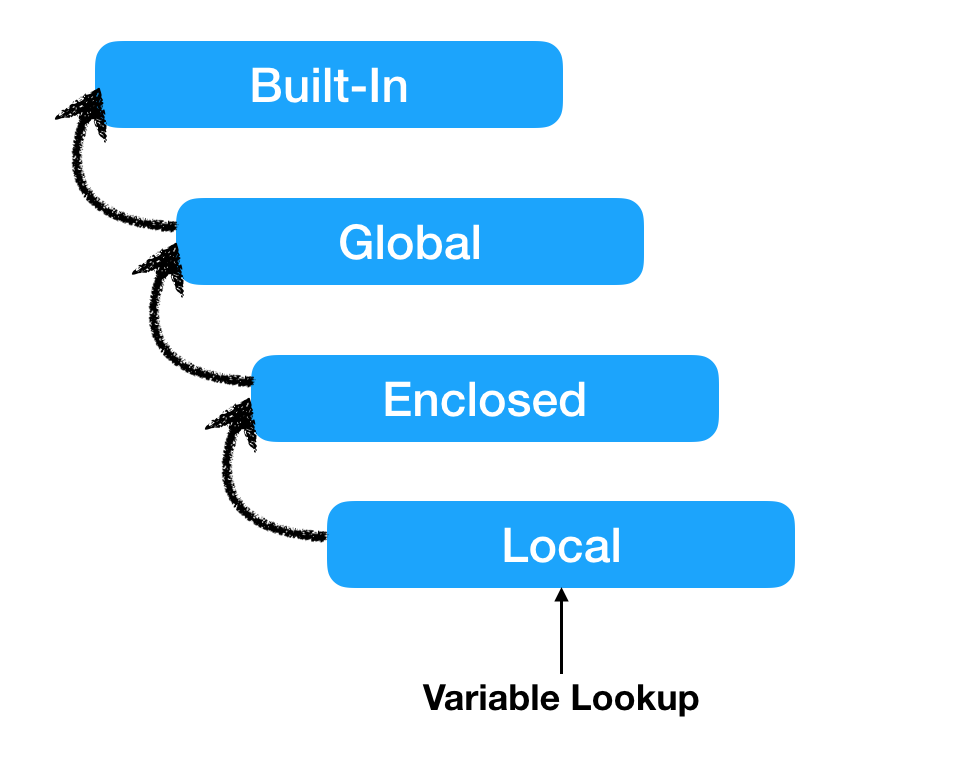# Python Namespace and Variable Scope Resolution (LEGB)In this tutorial, we will learn about Python namespace, the scope of a variable and the rules for variable scope resolution.

## What is Python Namespace?

Python namespaces are containers to map names to objects. In Python, everything is an object and we specify a name to the object so that we can access it later on.

You can think of namespace as a dictionary of key-value pairs where the key is the variable name and the value is the object associated with it.

```namespace = {"name1":object1, "name2":object2}
```

In Python, multiple independent namespaces can exist at the same time. The variable names can be reused in these namespaces.

```function_namespace = {"name1":object1, "name2":object2}

for_loop_namespace = {"name1":object3, "name2":object4}
```

Let’s look at a simple example where we have multiple namespaces.

## Python Namespace Types and Lifecycle

Python namespaces can be divided into four types.

1. Local Namespace: A function, for-loop, try-except block are some examples of a local namespace. The local namespace is deleted when the function or the code block finishes its execution.
2. Enclosed Namespace: When a function is defined inside a function, it creates an enclosed namespace. Its lifecycle is the same as the local namespace.
3. Global Namespace: it belongs to the python script or the current module. The global namespace for a module is created when the module definition is read. Generally, module namespaces also last until the interpreter quits.
4. Built-in Namespace: The built-in namespace is created when the Python interpreter starts up and it’s never deleted.

## Python Variable Scope

Python variable scope defines the hierarchy in which we search for a variable. For example, in the above program, the variables are present in different namespaces. When we want to access a variable value by its name, it is searched in the namespace hierarchy.

## Python Variable Scope Resolution Rules (LEGB)

Python variables are searched in the following order of namespaces.

```Local -> Enclosed -> Global -> Built-in
```

This is also called LEGB rule for variable scope resolution.

If a name is not found in the namespace hierarchy, NameError is raised.

When we create an object or import a module, we create a separate namespace for them. We can access their variables using the dot operator.

```>>> import math
>>>
>>> import numpy
>>>
>>> print(math.pi)
3.141592653589793
>>> print(numpy.pi)
3.141592653589793
>>>
>>> obj = object()
>>> print(obj.__doc__)
The most base type
>>>
```

Let’s look at an example of variable scope resolution involving all the namespaces.

```x = 10

print(f'x is {x}')

def outer():
x = 20
print(f'x is {x}')

def inner():
x = 30
print(f'x is {x}')
print(len("abc"))

inner()

outer()
```